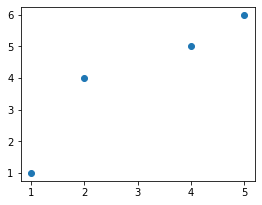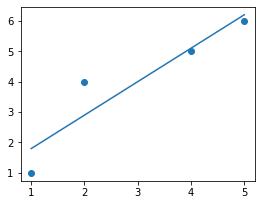search
Search
Publish
Guest 0reps
Thanks for the thanks!
close
account_circle
Profile
exit_to_app
Sign out
search
keyboard_voice
close
Searching Tips
Search for a recipe: "Creating a table in MySQL"
Search for an API documentation: "@append"
Search for code: "!dataframe"
Apply a tag filter: "#python"
Useful Shortcuts
/ to open search panel
Esc to close search panel
to navigate between search results
d to clear all current filters
Enter to expand content previewDoc SearchCode Search BetaSORRY NOTHING FOUND!
mic
Start speaking...Voice search is only supported in Safari and Chrome.
Shrink
Navigate to
A
A
share
thumb_up_alt
bookmark
arrow_backShareTwitterFacebook

# Performing linear regression in NumPy

Programming
chevron_right
Python
chevron_right
NumPy
chevron_right
Cookbooks
schedule Mar 10, 2022
Last updated
local_offer PythonNumPy
Tags
expand_more

Linear regression, in essence, is about computing the line of best fit given some data points. We can use NumPy's `polyfit(~)` method to find this line of best fit easily.

Here's some toy dataset, which we will visualize using `matplotlib`:

``` import matplotlib.pyplot as pltx = [1,2,4,5]y = [1,4,5,6]plt.scatter(x, y)plt.show() ```

This produces the following:Our goal is to fit a linear line through the data points. We do this by using Numpy's `polyfit(~)` method:

``` fitted_coeff = np.polyfit(x, y, deg=1)print(fitted_coeff) array([1.1, 0.7]) ```

Here, the `deg=1` just means that we want to fit a degree 1 polynomial, that is, the line `y=mx+b`. The returned values are the coefficients of the line of best fit, and the first value is the coefficient of the largest degree, that is, `m=1.1` and `b=0.7`.

Let's graph the line of best fit to see how good it is:

``` x = [1,2,4,5]y = [1,4,5,6]plt.scatter(x, y)line_x = np.linspace(1, 5, 100)plt.plot(line_x, line_x * fitted_coeff + fitted_coeff)plt.show() ```

This produces the following:This looks like a solid fit.

WARNING

Numpy's `polyfit(~)` method is just for computing the line of best fit

Numpy's `polyfit(~)` method does not compute any statistical measures like residuals and p-values. This method is only used when you just need the coefficients - nothing more, nothing less.

To perform linear regression at a more comprehensive level, use `scipy.stats.linregress`.